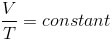﻿ Gas Laws
﻿

## Kelvin Temprerature

T: temperature in Kelvin scale, t: temperature in Celsius scale## Equation Of State

p: pressure(P, Pascal), V: volume(m3), n: number of moles of the gas(mol), R: universal gas constant, T: Kelvin temperature of the gas (K), k: Boltzmann’s constant, N: number of molecules in the gas.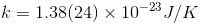## Ideal Gas Law## Boyle's Law For Pressure

If the temperature of the gas is constant## Gay-Lussac's Law Of Pressure-Temperature

If tha volume on a gas is constant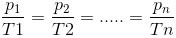P: pressure of gas at temperature t, po; pressure at 0 celsius, γ: factor when volume is constant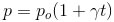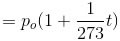Example when t=0 kelvin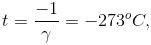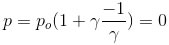## Charle's Law Of Volumes

If the pressure on a gas is constant